Author: Neo Ge

The original article was published here in Chinese. Since it's difficult to translate technical concepts from one language to another perfectly. Please feel free to correct me if I am mistaken. Now let's watch a 2-minute video I really enjoyed.

.   .   .

Recently, a lot of friends asked me about quantum computers. The biggest worry is that quantum computing will use its supercomputing power to make cryptocurrencies no longer encrypted and even destroy blockchain networks. Let me start with the answer: No. But to understand this, it is necessary to understand what quantum computing is and the cryptographic algorithm of blockchain. I'd like to appraise the technical support provided by Junkai Zeng. He is not only my former classmate but also a Ph.D. in Physics from Virginia Tech. His research direction is quantum computing. He is currently working in a quantum company invested by a Sequoia in LA.

# Quantum Computing

To understand quantum computing, we must understand what classical computing is. We need to know that there is a bottleneck encountered in the development of classic computers called Moores Law, that is, the number of transistors that can be accommodated on integrated circuits will double every two years. This results in the transistor becoming smaller and smaller. When it reaches about 2nm because the transistor at the 2nm level can only accommodate ten atoms, the behavior of electrons will no longer obey traditional semiconductor theory, but follow quantum mechanics. As the integration of transistors increases, the heat generated by the chip will become higher and higher. These adverse effects have severely hindered the development of classic computers. Under such worries, people came up with solutions such as photonic computers, biological computers, and quantum computers.Moore's Law — The number of transistors on integrated circuit chips (1971–2018)

# Why is quantum computing so valued?

First of all, we need to know that the operation of a classic computer is completed by a logic circuit. Binary 1s and 0s represent logical operations in the computer, also known as Boolean algebra. 1 represents true (high level), 0 represents false (low level). The most common logical operations are AND, OR, and NOT. For example, true AND true results are true, true AND false results are false; NOT true is false; NOT false is true. These operations are completed through logic gates, which can be reversible or irreversible. For example, the NOT gate inputs a 1 (true), and the output is a 0 (false), so the NOT gate is reversible because we can invert the input 1 (true) by outputting 0 (false). But if the output of an AND gate is 0 (false), then the input bits have three possibilities, namely 00, 01, and 10. We cannot explicitly map back to the input one-to-one, so the AND gate is an irreversible gate, where the two-bit information becomes one-bit information after passing through the AND gate, one bit of information is lost here.

# Landauer's Principle

In 1961, IBM engineer Rolf Landauer discovered that every bit of information erased required a thermodynamic cost, that is, at least kTln2 of heat was generated, while writing information did not require a thermodynamic cost. Where k is the Boltzmann constant, and T is the ambient temperature. This is the famous Landauer's Principle, through which we know that information also has physical properties. The classic computers are full of irreversible operations so that classic computers will generate more and more heat. This is Landauer's conclusion when thinking about Maxwell's demon. Because processing information is costly, and the demon's memory cannot be infinite, it will always delete some of the memory and cause the entropy to increase, so the demon cannot exist.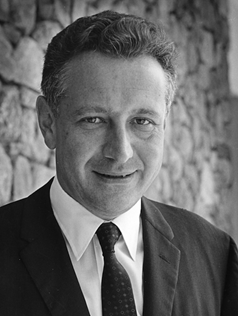Rolf Landauer

So people think that if the irreversible operation in a classic computer is converted into a reversible operation, then the problem will not be solved. In theory, an operation called unitary transformation — a processing method for quantum states, placed on a classical computer could solve the problem of heat dissipation. However, although classical computers are good at differential (simulating classical physical systems), it is nearly impossible for classical computers to simulate quantum physics systems. So later, Feynman and other physicists proposed to convert classical bits into qubits directly and use quantum to simulate quantum systems. (What we heard in the video at the beginning of the article was Richard Feynman's voice).

# Quantum's natural advantage — parallel computing

In classic computers, we use binary to improve computational efficiency. If you want a classic computer to calculate 100 + 100, you need to convert the decimal 100 to the binary 1100100 first. In a classic computer, you need to use seven transistors in different states to represent binary 1100100. Generally speaking, a transistor is a bit, and can only represent 1 or 0 (high or low). Quantum computers are different, where one qubit is a two-state system by default. A two-state system is like the spin of an electron or the polarization of a photon. The most popular explanation is Schrödinger's cat. Schrödinger's cat is in a superposition state of dead and alive from the perspective of quantum mechanics when it is not observed, which follows the principle of superposition of quantum states. If we replace the transistor in the classic computer with a Schrödinger's cat, one Schrödinger's cat can represent 1 and 0 at the same time, instead of 1 or 0 in the classic computer. Two Schrodinger cats can represent four states of 00, 01, 10, and 11, and two bits in a classical computer can only take one of these four states. So for n bits, classical bits can only represent 1 number, and qubits can theoretically represent 2ⁿ numbers at the same time, which is the biggest difference between classical computers and quantum computers. This 2ⁿ style exponentially growing temptation has made people very interested in quantum computers, which is the benefit of natural parallel computing.Schrödinger's catHow to implement a quantum computer

# How to implement a quantum computer

## Question 1: What can be used as a qubit?

From the perspective of a physicist, in theory, two-level quantum systems can be used as qubits. For example, when the electron spin is not measured, it is in a superimposed state that is both up and down. Once measured, an eigenstate that is either up or down will appear.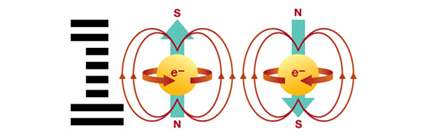Electronic spin

## Question 2: What algorithm do we use in quantum computers?

Since the algorithm is the soul of the computer, and the classic algorithm is only applicable to the classic bit, even if the quantum chip is implemented really well, the classic algorithm cannot be used on the quantum chip. In 1994, Peter Shor of Bell Labs published the Shor's algorithm that can perform the prime factorization of large numbers.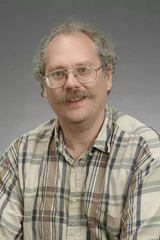Peter Shor

The prime factorization problem is an NP (nondeterministic polynomial time) problem, that is, its complexity cannot be completed in polynomial time in classical algorithms. The complexity of a general algorithm for prime factorization is O or eⁿ, where n refers to the number of bits represented in binary. It takes about 150,000 years to use the fastest classical supercomputing to perform a 300-digit (decimal) prime factorization. This is why the prime factorization of large numbers can be used for encryption because it is almost impossible to use classical computer brute force to calculate which two prime numbers are multiplied to decrypt. But the Shor's algorithm can reduce the complexity to (logN)³, which means that Shor's algorithm turns an NP problem into a P-type problem. Using the Shor's algorithm to perform 300-digit prime factorization also requires less than one minute in theory. Also, Shor has solved a problem that has troubled physicists for more than ten years. If the Shor algorithm is used to calculate qubits, the result is still a bunch of qubits. We need to measure the results. However, Schrödinger's cat tells us that the probability of measuring a cat's life and death accounts for 50% each, so the theoretically measured results are random. Shor tells us that by using Shor's algorithm, the required data will perform constructive interference, and the unnecessary data will perform destructive interference. The probability of measuring the correct result at the end is 1. Since the confidential data of banks and governments are encrypted based on the prime number RSA algorithm, quantum computing has aroused strong interest from governments around the world since the release of the Shor's algorithm in 1994. Even the DARPA (US Defense Advanced Research Projects Agency) has joined the fight (DARPA has proposed and invested in the Internet, Unix system and GPS, etc.).

In 2001, IBM's Chinese-American researcher Isaac Zhuang and their research team demonstrated the Shor's algorithm by nuclear magnetic resonance, that is, a molecule composed of five fluorine atoms and two carbon atoms, using the nuclear spin of each atom as a qubit. 7-qubit is equivalent to 2⁷, which is 128 classic bits. Using this mini-quantum computer, the prime factorization of 15 was performed using Shor's algorithm, and the results were 3 and 5, which successfully proved that Shor's algorithm is feasible. It was later shown that Shor's algorithm could also be used to solve discrete logarithm problems. Quantum computer algorithms include Grover, QAOA, and VQE algorithms in addition to the Shor's algorithm. We will get into the Grover algorithm later.

## Question 3: How to maintain quantum coherence?

The coherence of the quantum is challenging to maintain. Even if the quantum in the superposition state is not measured, it will entangle quantum with the surrounding environment and then become a definite state, that is, become a classic, which is commonly known as wave function collapse. The process is called quantum decoherence. To resist the impact of quantum decoherence, we have two principal methods: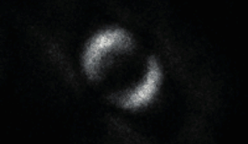Quantum entanglement

1. Quantum error correction (QEC), similar to the classic computer, at the expense of resources, such as using eight qubits for one, it's alright if there are one or two decoherences, correcting a few decoherent quanta. There are two problems with using error correction codes: one is that the decoherent of each step of quantum computing must be lower than a certain ratio (like 1%) so that the error can be corrected; the other is that the error correction code requires a large number of physical layers Qubits to encode a logical level of qubits, these have brought great obstacles to the realization of quantum computing at the hardware level.

2. Handle the quantum system with better technology: because of some properties of quantum mechanics, even if the same operation is achieved, controlling the qubits in different ways on the physical level will bring very different fidelity. By using better methods to optimize these operations, the error rate can be controlled below the threshold of quantum error correction, paving the way for more general quantum error correction.

So far, quantum computers have no problems in theory. As for the physical level, how to manufacture quantum chips, and what are they made of? This is the main research direction of the major institutions at present, to ensure that the qubits are integrated while maintaining coherence. Typical hardware implementation solutions for implementing qubits mainly include optical systems, semiconductor quantum dots, superconducting circuits, cold atoms, ion wells, and so on. Industry research institutes such as Google, IBM, and Alibaba Institute are dedicated to implementing quantum chips through superconducting circuits. In September last year, Google announced the successful use of a processor containing 53 effective qubits to achieve Quantum Supremacy. It should be pointed out that "Quantum Supremacy" refers to the achievement of certain problems that quantum computing can solve but classic computers cannot, the "quantum" supremacy over "classic", which is new technology supremacy over the old technology. It's not a particular enterprise or research institution leading the world, so there is supremacy over other enterprises and research institutions.

# Blockchain Encryption Algorithm

I will be using Bitcoin as an example to introduce the encryption algorithm of the blockchain. There are two types (three kinds) of encryption algorithms in Bitcoin, namely the elliptic curve algorithm (ECA), SHA256 hash algorithm, and RIPEMD160 hash algorithm. The general process is as follows:

`Private key -> ECA -> public key -> SHA256 -> RIPEMD -> SHA256 -> SHA256 -> address`

The one-way arrow means that the algorithm in the classic calculation is irreversible, the public key cannot be reversed from the address, and the public key cannot be reversed from the private key. Since quantum computing is to convert the irreversible in classical computing into reversible, to facilitate the explanation, we start to investigate backward from the address.

# Hash algorithms

Among the two hash algorithms used by Bitcoin, SHA256 is an algorithm subdivided under SHA-2. It is a cryptographic hash function algorithm standard developed by the National Security Agency; RIPEMD (RIPE Message Digest) is also a hash function. For symmetric encryption and hash functions, there are quantum attacks, but they are less dangerous. The Grover algorithm can only reduce the difficulty of 2²⁵⁶ under classical calculation to 2¹²⁸, which is still astronomical, and from the public key to the address, it needs to perform one time of RIPEMD and three times of SHA256 hash operations, so we don't have to worry until there is a subversive quantum algorithm for symmetric encryption.

Since Bitcoin mining uses the SHA256 algorithm if a quantum algorithm that is far superior to the Grover algorithm comes out in the future, and as time goes by, the quantum computer becomes faster, and the price becomes lower, then the quantum computer will indeed surpass the classic computer in Bitcoin mining. But this process is like from CPU mining to GPU mining to ASIC mining, we don't have to worry about it at all.

# Elliptic Curve Algorithm (ECA)

There is only one barrier to reversely push back the private key from the public key — Elliptic curve cryptography (ECC), which is an algorithm for establishing public key encryption, that is, asymmetric encryption. From a mathematical point of view, an elliptic curve can be expressed as follows:Bitcoin's elliptic curve digital signature algorithm (ECDSA) based on the secp256k1 curve is one of the elliptic curves. Since the elliptic curve algorithm depends on the difficult problem of discrete logarithms and the above-mentioned Shor's algorithm can be used to solve the discrete logarithm. Theoretically, a quantum computer with a certain effective qubit will pose a threat to Bitcoin's elliptic curve algorithms. However, the security of the secp256k1 curve is 2¹²⁸, so even if the Shor's algorithm reduces its complexity to 128³, the quantum computer that attacks the secp256k1 curve should theoretically have at least 1,500 logical qubits. Considering the use of quantum error correction, the physical qubits actually required will be much higher than this number. The largest general-purpose quantum computer announced by Google in September last year has only 53 physical qubits. Its error rate is extremely high, and it can only be operated under laboratory conditions close to absolute zero. At the same time, the superconducting chips used by Google naturally have many problems in scalability, so how to add more qubits on the basis of maintaining controllability will be a huge challenge in the foreseeable future. Although it is not possible to predict precisely how fast quantum computing will develop, it is expected that Bitcoin's 256-bit ECDSA key will be secure at least by 2040.

Bitcoin itself has some built-in anti-quantum qualities. If you have a good Bitcoin usage habit, that is, a wallet address is only used once, then your public key will be broadcast to the entire network only when you spend Bitcoin. At this time, the quantum computer will only have a very short time to crack your private key, that is, the period from the time the transaction is sent until the transaction information is added to the block.

Let's assume that you don't have a good bitcoin usage habit, quantum computers have developed by leaps and bounds, and all common public-key algorithms have been destroyed. Bitcoin also has a killer tool, which is to upgrade its encryption algorithm. As we all know, if a technical method can crack a password, it will inevitably also be able to create a password that cannot be cracked. Currently, among public key encryption algorithms for quantum security, Bitcoin experts tend to prefer cryptosystem based on Lamport signatures. Although the Lamport signatures are very fast to compute, there are two main disadvantages:

1. The signature would be very large, at least a few kB (40–170 times larger than it is now), which will be very detrimental to the overall scalability of Bitcoin.

2. When creating each keypair, you need to set the finite maximum number of valid signatures with this key. If the signature exceeds this number, it will no longer be safe. But if you only use each address once, this should not be a big problem.

Also, in the research of post-quantum cryptography by cryptographers, Supersingular Isogeny Diffie–Hellman(SIDH) Key Exchange is also expected to replace the current conventional Elliptic Curve Diffie-Hellman (ECDH) Key Exchange. Cryptography based on high-dimensional vector space, that is, lattice-based cryptography will also increase the difficulty of deciphering by another cosmic magnitude, which is enough to fight quantum computing. The new public key algorithm will be added to Bitcoin as a soft fork, and Bitcoin users only need to send their Bitcoin to a newly created address to achieve quantum security.

# Cryptography and Computer Science

When we study the challenges of quantum computers for blockchain, it is difficult not to think about the relationship between cryptography and computer science. The facts of history are always strikingly similar that people develop computers to break ciphers.

During World War II, Alan Turing, the father of computer science and artificial intelligence, was employed by the Royal Navy to decipher German military secret codes. At that time, the Nazi military had a complex and sophisticated communication security system-the Enigma cipher machine. It consists of a series of rotors that are continually changing at random, and its complexity is as high as 10 quadrillion, which is extremely difficult to overcome with the deciphering methods at the time. Turing used his deciphering machine "bombe" to decipher Enigma first, and then deciphered almost all levels of communication encryption systems used by the Nazi military, including the Tunny ciphers. This made the Allied forces well aware of German military command and planning and helped the Allied forces defeat the Nazi Germans at least two years in advance, ending the Second World War.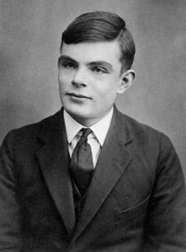Alan Turing

Turing proposes a mathematical logic machine that abstracts human computing behavior, namely the Turing machine. Later, the father of modern computers, Von Neumann, used the Von Neumann structure to complete the Turing machine. The Turing test named after him is widely used as a test method for determining whether a machine is intelligent. Turing's brilliant achievements in mathematics and logic also set a grand blueprint for the development of computers and the entire digital era. In recognition of his pioneering contribution, the Nobel Prize in the computer industry named after him — Turing Award was officially established in 1966 as the highest award in this field.

For the coming quantum era, as blockchain practitioners, we should welcome it with awe and expectation. The value of quantum computers lies in solving typical NPC problems and other problems such as the Three-body problem, Seven Bridges of Königsberg, protein folding, earthquake prediction, and weather forecasting. A quantum computer is not a panacea, it cannot replace a classic computer.

.  .  .

## References

 Martin Roetteler, Michael Naehrig, Krysta M. Svore, and Kristin Lauter. "Quantum Resource Estimates for Computing Elliptic Curve Discrete Logarithms" Microsoft Research, USA (2017): 1706.06752

 Matthew Amy, Olivia Di Matteo, Vlad Gheorghiu, Michele Mosca, Alex Parent, and John Schanck. "Estimating the cost of generic quantum pre-image attacks on SHA-2 and SHA-3" Quantum Physics (2016): 1603.09383

 孙昌璞, 全海涛. "麦克斯韦妖与信息处理的物理极限" 物理·42卷11期 (2013): 10.7693

 Craig Gentry. "Fully Homomorphic Encryption Using Ideal Lattices" Stanford University and IBM Watson (2009): cs.cmu.edu

 Frank Arute, Kunal Arya, Ryan Babbush, Dave Bacon, Joseph C. Bardin, Rami Barends et al. "Quantum supremacy using a programmable superconducting processor" Nature 574, no. 7779 (2019): 505–510

 I. Stewart, D. Ilie, A. Zamyatin, S.Werner, M. F. Torshizi and W. J. Knottenbelt. "Committing to quantum resistance: a slow defence for Bitcoin against a fast quantum computing attack" Royal Society Open Science 5, no. 6 (2018): 180410

 Divesh Aggarwal, Gavin K. Brennen, Troy Lee, Miklos Santha, and Marco Tomamichel. "Quantum attacks on Bitcoin, and how to protect against them" Quantum Physics (2017): 1710.10377

 Zhe Zhou. "MommyTalk" YouTube (2019)